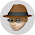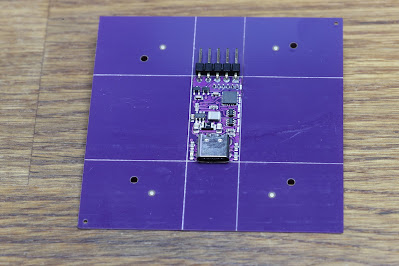## 2013-12-24

### 3D maths objects

I have been having some fun with 3D objects created from mathematical formulae. A couple of good examples are the trefoil knot and the torus knot.

The starting point for both was wikipedia which has the 3D Cartesian parametric equations for this. Lets look at the trefoil knot first.

x = sin t +2 sin 2t
t = cos t - 2 cos 2t
z = - sin 3t

Using openscad I was able to make a simple module that does a translate based on this. But I found it looked better if I adjust the height (z) a bit, using h=4/3, and q=2/3.

translate([sin(t)+2*sin(2*t),cos(t)-2*cos(2*t),-h*sin(3*t)])
sphere(q);

A simple way to use this was to make a loop, e.g. for(t=[0:1:359]) can in that I can make a hull of the function f(t) and f(t+1). This creates two spheres and joins them and does that for each step. This works, and creates a trefoil knot that is based on a circular cross section tube. It is slow, and you have to make the sphere have a lot of segments to appear round.

So I decided I could have more fun if I pushed a shape along the path. This means that I make a shape at each point, such as a triangle, square, pentagon, or even circle. The problem is that at each point I need to point the shape in the direction we are going. This means differentiating the original parametric function in three dimensions.

What I ended up with is :-

translate([sin(t)+2*sin(2*t),cos(t)-2*cos(2*t),-h*sin(3*t)])
rotate([0,90+atan2(1,-3*h*cos(3*t)),atan2(4*sin(2*t)-sin(t),cos(t)+4*cos(2*t))])
rotate([0,90,0])
rotate([0,0,(\$fn%2)?3*(t-30)/\$fn:180/\$fn])
cylinder(r1=q,r2=0,h=0.01);

The first part is the position based on t. Then I rotate to face the direction based on a differential of the original function, and then turn 90 degrees to face the direction. The then rotate the shape which is based on using \$fn in openscad to make a triangle, square, pentagon, or so on. I actually rotate it so that it has a flat base on the bottom for easy printing but also, for odd numbered shapes, I rotate along the path to ensure all three points that touch the base are also flat as well as the top of the shape, and making it mobius so that the shape has a twist where it connects at the end. Finally a cylinder with small height and r2=0 is the best I could come up with to make a cross section to form the hull for each step. Using circle() did not work as it makes a cylinder with height 1.

The result is this. Using a pentagon (\$fn=5).

The print looks pretty cool, and prints well on a Makerbot.

Having worked out how to do this, I created the same system for a torus knot. The formulae is actually very simple.

x = r cos pt
y = r sin pt
z = - sin qt

Where r is cos qt + o, and o is at least 2, but works well with 3. Basically o is the size of the torus. This all led to the openscad :-

translate([(cos(q*t)+o)*cos(p*t),(cos(q*t)+o)*sin(p*t),-sin(q*t)])
rotate([0,90-atan2(-q,-q*cos(q*t)),atan2(p*cos(p*t)*(cos(q*t)+o)-q*sin(p*t)*sin(q*t),-q*cos(p*t)*sin(q*t)-p*sin(p*t)*(cos(q*t)+o))])
rotate([0,90,0])
rotate([0,0,(\$fn%2)?q*(t-90/q)/\$fn:180/\$fn])
cylinder(r1=r,r2=0,h=0.01);

Which creates for \$fn=5, p=3, q=7, o=3 :-

I have put on thingiverse, trefoil and torus knots.

But this does create a working principle for any sorts of 3D knot shapes.

1.Are the ridges (ribs?) on the plastic model the same ones on the render? If so, is there a nice easy way of smoothing the knot out?

1.I can do finer slices, etc. And using a pentagon creates edges but that too could be made closer to a circle. Lots of scope to tiff up. Ultimately you are slicing and printing, e.g. .2mm or .1mm is typical at present.

2.How does it 'print'? I'm trying to imagine the movement of the print head to generate these interlocked elements.

Comments are moderated purely to filter out obvious spam, but it means they may not show immediately.

### Assembled PCBs from China

I have been using JLCPCB to make PCBs for a while. This is for some of the smaller PCB projects at A&A ( here ), rather than the big pro...